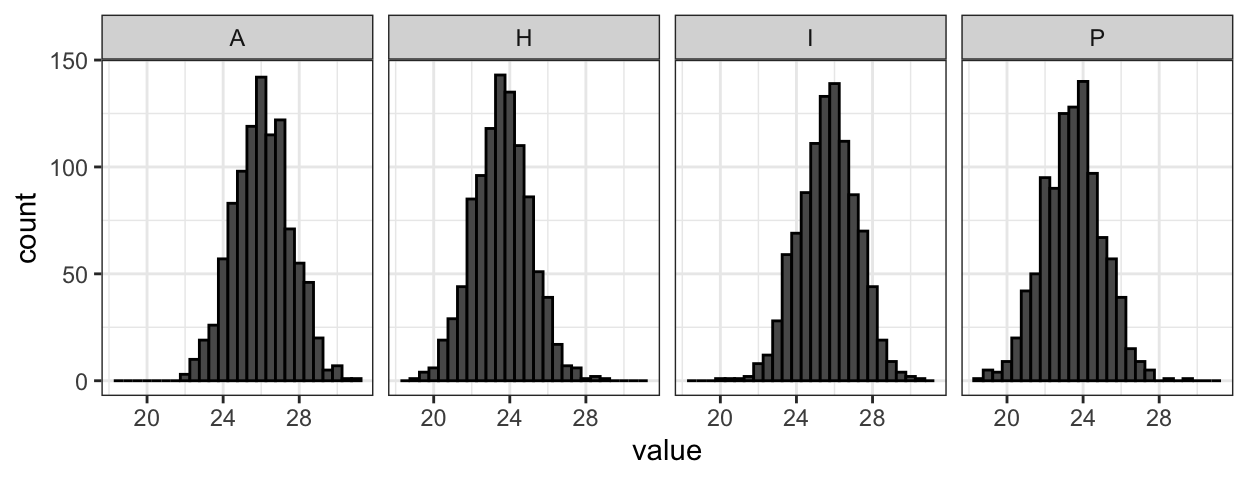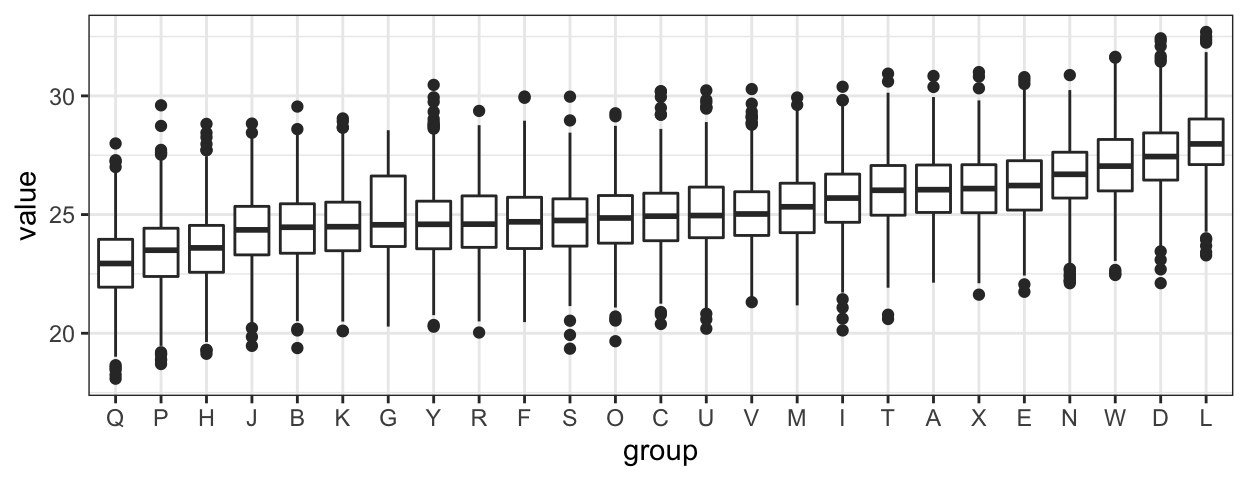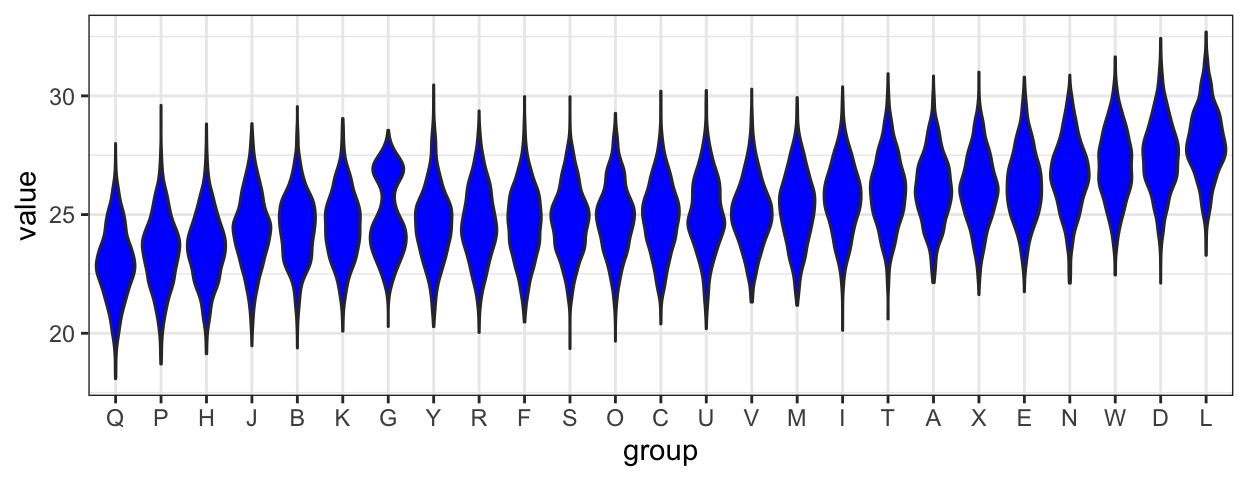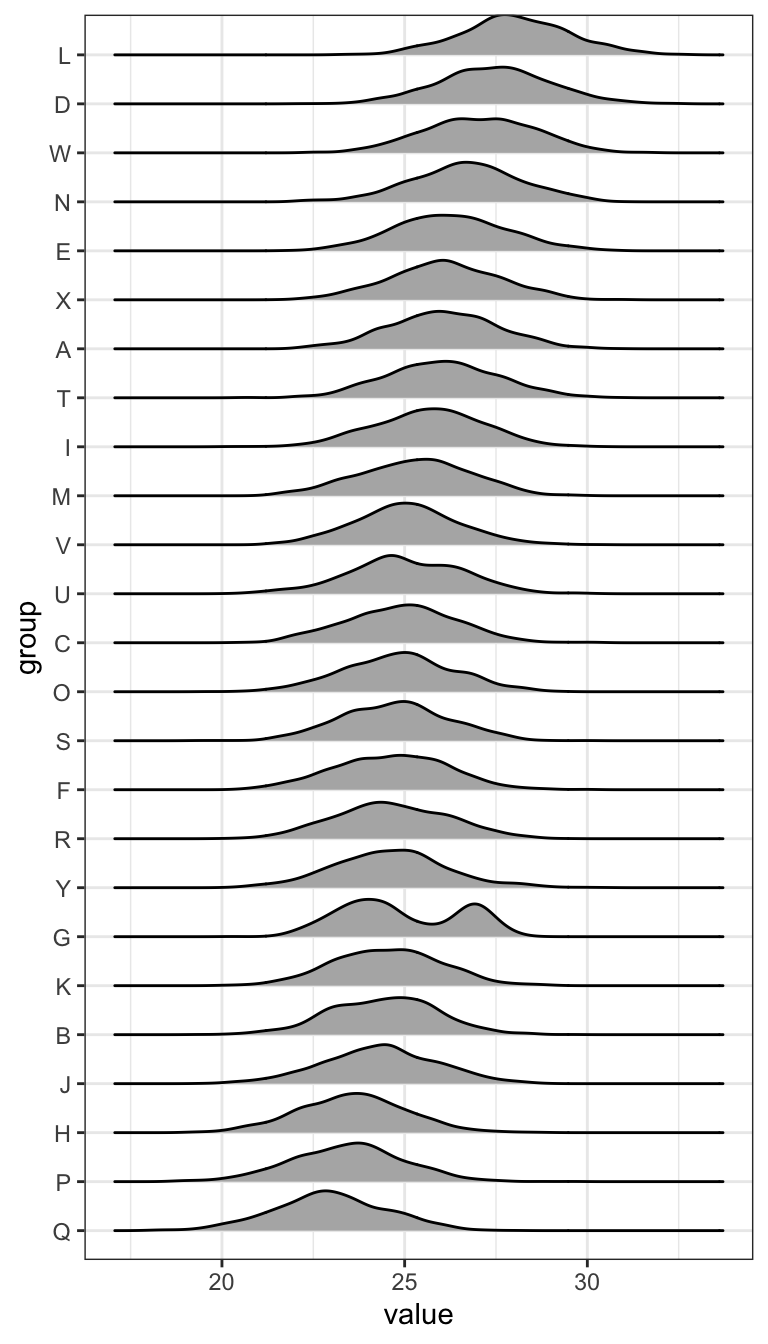# The joy of no more violin plots

Rafael Irizarry
2017-07-13

Update: The ggjoy package has been deprecated. The ggridges CRAN package provides the same functionality.

I dislike violin plots because they look like Christmas ornaments.It’s a pet peeve but there is somewhat of a practical reason as well. To demonstrate I created a dataset called `dat` that contains an outcome `value` from 25 different groups.

One of the first steps I take when analyzing data is to look at the distribution of my data. If there are groups, I like to stratify and look at the distributions. Histograms and smooth density estimates are my favorite tools for visualizing distributions. I have 25 groups in this data so I would start by quickly looking at a random subset:

``````dat %>% filter(group %in% c("A","H","I","P")) %>%
ggplot(aes(value)) +
geom_histogram(binwidth = 0.5, color="black") +
facet_grid(.~group)``````This plot shows me that the data looks normally distributed. To see how the groups compare to each other, a first step is to look at boxplots with groups reordered by their median value.

``````dat %>% mutate(group = reorder(group, value, median)) %>%
ggplot(aes(group, value)) + geom_boxplot()``````If you look close enough you note that group G looks a bit different: larger variance. But by summarizing into only five numbers, the boxplot misses an important characteristic of the data. Violin plots let you see the distributions rather than these five points:

``````dat %>% mutate(group = reorder(group, value, median)) %>%
ggplot(aes(group, value)) + geom_violin(fill = "blue")``````Do you see it? If you look closely, group G appears to be bimodal. But why is it hard for me to see?

1. Adding a mirror image of the density to make them resemble boxplots in some manner makes them look like Christmas ornaments and distracts me.
2. I am used to looking at histograms and densities with the variable in the x-axis not the y-axis.

I therefore prefer to stack histograms or density estimators vertically. So I was happy to learn about the ggjoy package that let’s you make such a plot in one line of code and produces a much more aesthetically pleasing plot than stacking histograms using, for example, facets.

``````library(ggjoy)
dat %>% mutate(group = reorder(group, value, median)) %>%
ggplot(aes(x=value, y=group, height=..density..)) +
geom_joy(scale=0.85)``````Note how quickly we notice the bimodal group. I am hoping people start using joy plots instead of violin plots. To install and try out the package use this:

``````library(devtools)
install_github("clauswilke/ggjoy")``````